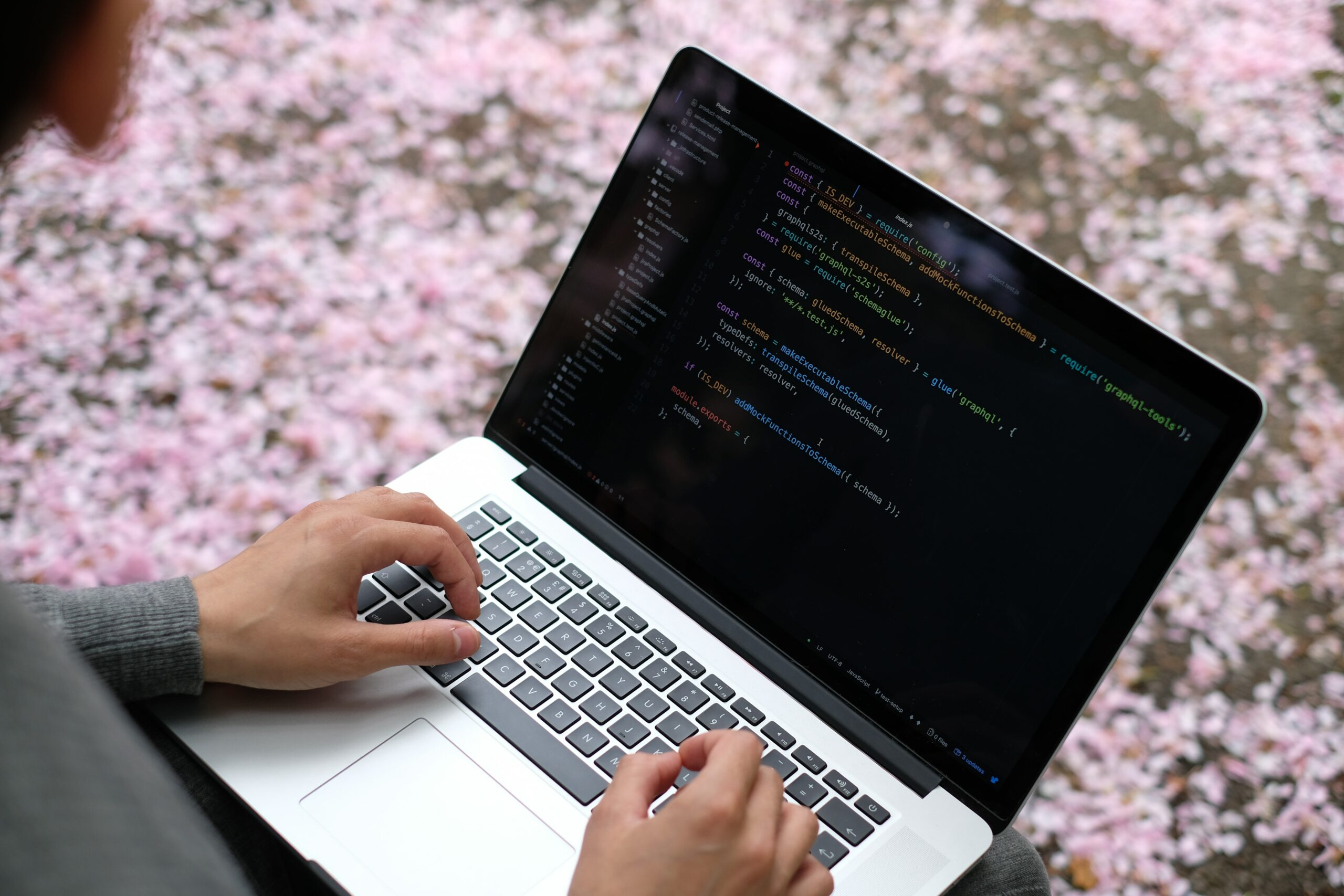# When All Else Fails, Just Write a ProgramAssociated Subjects:

Math

In Mrs. Ostaff’s Algebra I class, students were given a challenge word problem to figure out.

Here’s the problem:

Sarah, Gwen, and Emma were playing a game with a box of 40 counters – they were not using all of them. They each had a small pile of counters in front of them. All at the same time, Sarah passed a third of her counters to Gwen, Gwen passed a quarter of her counters to Emma, and Emma passed a fifth of her counters to Sarah. They all passed on more than one counter. After this they all had the same number of counters. How many could each of them have started with?

Mrs. Ostaff said she intended to have students figure out the answer by using separate equations, or by using manipulatives, but student Caden K. wrote a program instead. Mrs. Ostaff said this was one of the coolest and most creative answers she’s ever received. Check Caden’s program out below!

First, I wrote the following equation which is really just the sentences, but turned mathematical. I’ve turned each person’s collection of tokens into the first letter in their name. S/3+G*3/4==G/4+E*4/5==E/5+S*2/3. I then wrote a Python program to solve it for me. =D. I asked, and Mrs. Ostaff said it was allowed. Below is the program I wrote. You can copy and paste it into a Python Shell and run it, it should work. Basically though, I told it to cypher through all possibilities and try each one in the equation or check, I guess, that I wrote. I didn’t really need to assign each girl a list of their possibilities. I have them there because originally I was going to have the program only try multiples of the girl’s fraction that they gave away, because I knew it was divisible by that number since it says they could easily give a fraction of that portion. However, it wouldn’t save any time programming and it would barely save any time for the computer(since they’re so fast), so I scrapped that idea and had them try everything from one to forty, the upper limit. I actually got two solutions from this problem, but it said that each girl passed more than one token, so I went with the higher one. I’ve highlighted different sections of the program according to their purpose.

RED: List set up for what numbers to try

YELLOW: Where it tries numbers

GREEN: The actual checking if-statement

BLUE: Manual check where the user types the numbers themself.

After all this I took the numbers and did it on paper too, and that worked also, so, hurray, I did, the end.

```EMMA=[]
for i in range(1,40):
EMMA.append(i*5)
SARAH=[]
for i in range(1,40):
SARAH.append(i*3)
GWEN=[]
for i in range(1,40):
GWEN.append(i*4)

while 1==1:
for E in range(1,len(EMMA)):
for S in range(1, len(SARAH)):
for G in range(1,len(GWEN)):
if (E/5)+(S*(2/3))==(S/3)+(G*(3/4)) and (S/3)+(G*(3/4))==(G/4)+(E*(4/5))and(E/5)+(S*(2/3))==(G/4)+(E*(4/5)) and E+S+G<40:
print(“Emma: “+str(E))
print(“Sarah: “+str(S))
print(“Gwen: “+str(G))
print(“Ended”)
E=input(“Emma?”)
S=input(“Sarah?”)
G=input(“Gwen?”)
E=int(E)
S=int(S)
G=int(G)

if (E/5)+(S*(2/3))==(S/3)+(G*(3/4)) and (S/3)+(G*(3/4))==(G/4)+(E*(4/5))and(E/5)+(S*(2/3))==(G/4)+(E*(4/5)) and E+S+G<40:
print(“AWESOME IT WORKED!!!!”)
```

Share the love!